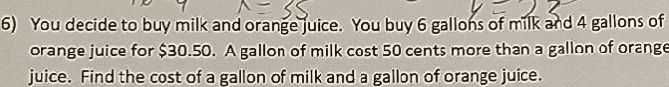### ¿Todavía tienes preguntas de matemáticas?

Pregunte a nuestros tutores expertos
Algebra
Pregunta6) You decide to buy milk and orange juice. You buy $$6$$ gallons of milk and $$4$$ gallons of orange juice for $$\ 30.50 .$$ A gallon of milk cost $$50$$ cents more than a gallon of orange juice. Find the cost of a gallon of milk and a gallon of orange juice.

$$6m+ 4o= 30.5\\m= 0.5+ o\\m= 3.25\\o= 2.75$$